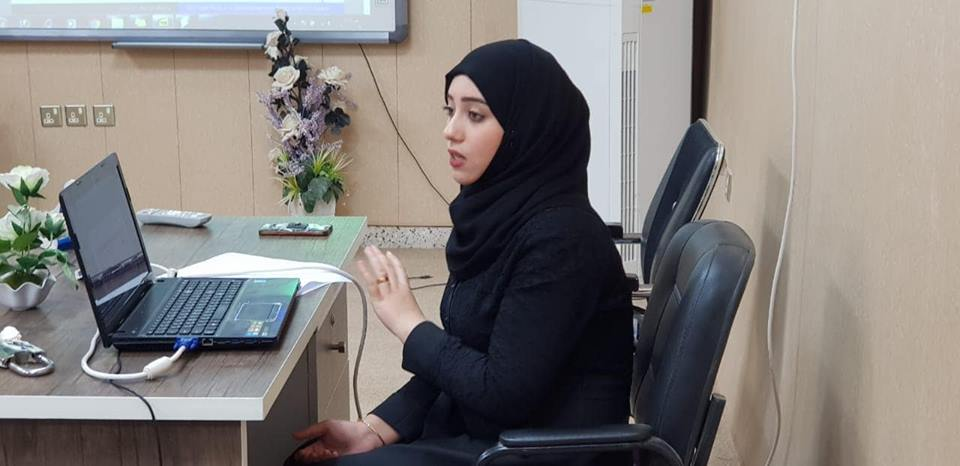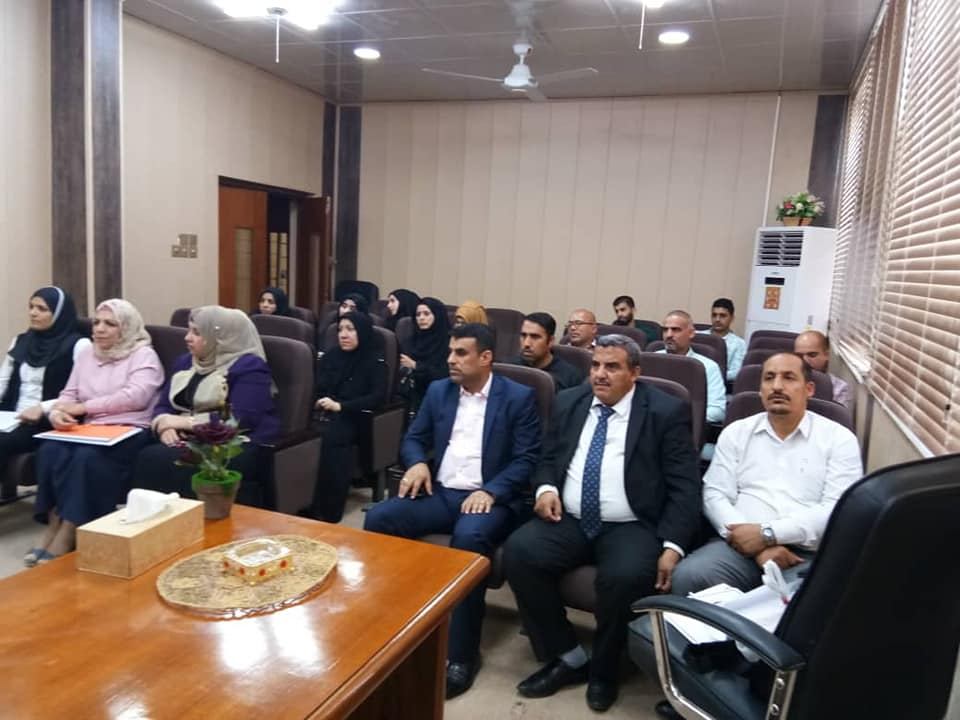## University of Basra discusses the Fixed Point in the metric space BThe Faculty of Science at the University of Basra discussed a seminar entitled "On the fixed point in the common and partial metric space B".

The main objective of the study is to give the introduction and the study of Coincidence and Common Fixed Points using the general condition B, which was presented by Majid Abbas et al. 2003.

In which it was combined with the B-rectangular (general) metric. Also, some new results were obtained using the concept of partial metric space and compound it with the metric space B Under the deflation condition that was introduced by LJ-B. Ciric.All rights reserved to Basrah University © 2018